Courses

# Doc: Introduction to Electrochemistry Class 12 Notes | EduRev

## JEE : Doc: Introduction to Electrochemistry Class 12 Notes | EduRev

The document Doc: Introduction to Electrochemistry Class 12 Notes | EduRev is a part of the JEE Course Chemistry for JEE.
All you need of JEE at this link: JEE

Key Concepts
Electrochemical Cells

An electrochemical cell consists of two electrodes (metallic conductors) in contact with an electrolyte (an ionic conductor).

An electrode and its electrolyte comprise an Electrode Compartment.

Electrochemical Cells can be classified as:

(i) Electrolytic Cells: Cells in which a non-spontaneous reaction is driven by an external source of current.

(ii) Galvanic Cells: Cells which produce electricity as a result of a spontaneous cell reaction .

Note: In a galvanic cell, cathode is positive with respect to anode.

In a electrolytic cell, anode is made positive with respect to cathode.

Galvanic Cell

This cell converts chemical energy into electrical energy.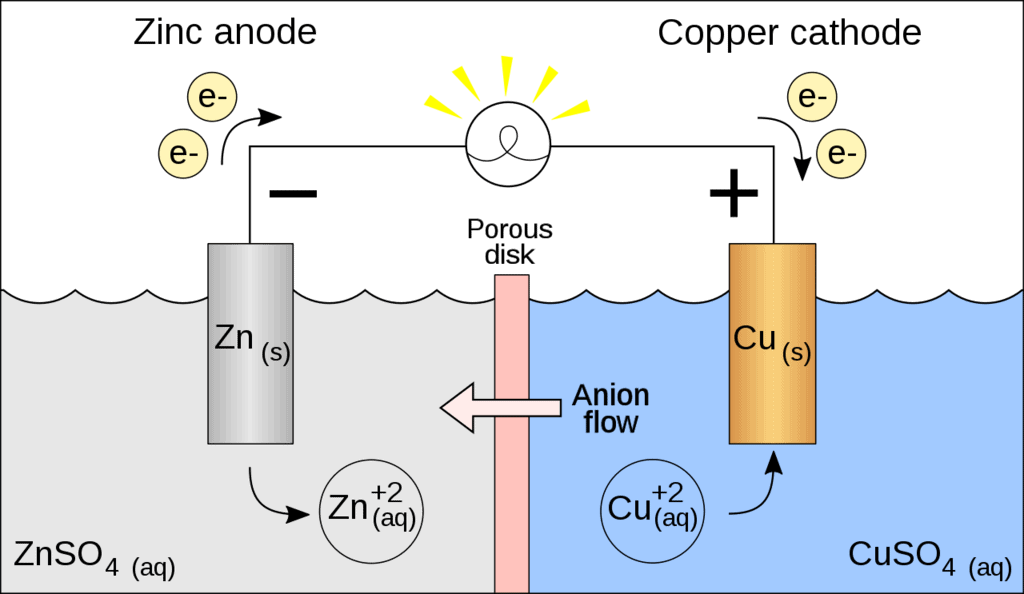Galvanic Cell

A galvanic cell is made up of two half cells i.e., anodic and cathodic. The cell reaction is of redox kind. Oxidation takes place at anode and reduction at cathode. It is also known as voltaic cell. It may be represented as shown in Fig. Zinc rod immersed in ZnSO4 behaves as anode and copper rod immersed in CuSO4 behaves as cathode.

Oxidation takes place at anode.

Zn → Zn2  + 2e- (loss of electron : oxidation)

Reduction takes place at cathode:

Cu2  + 2e- → Cu(gain of electron ; reduction)

Overall process: Zn(s) + Cu2+  → Cu(s) + Zn2+

In galvanic cell like Daniell cell: electrons flow from anode (zinc rod) to the cathode (copper rod) through external circuit; zinc dissolves as Zn2+  ; Cu2+  ion in the cathode cell picks up two electron and become deposited at cathode.

Representation of a cell (IUPAC Conventions):

Let us illustrate the convention taking the example of Daniel cell.

(i) Anodic half cell is written on left and cathodic half cell on right hand side.

Zn(s) |ZnSO4(sol)||CuSO4(sol)|Cu(s)

(ii) Two half cells are separated by double vertical lines: Double vertical lines indicate slat bridge or any type of porous partition.

(iii) EMF (electromotive force) may be written on the right hand side of the cell.

(iv) Single vertical lines indicate the phase separation between electrode and electrolyte solution.

Zn|Zn2+ ||Cu2+ |Cu

(v) Invert eletrodes are represented in the bracket

Zn|ZnSO4||H |H2,Pt

Electrochemical cell and Gibbs Energy of the Reaction

Electrical work done in one second is equal to electrical potential multiplied by total charge passed. If we want to obtain maximum work from a galvanic cell then charge has to be passed reversibly. The reversible work done by a galvanic cell is equal to decrease in its Gibbs energy and therefore if the emf of the cell is E and nF is the amount of charge passed and ΔrG is the Gibbs energy of the reaction, then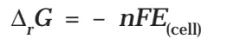It may be remembered that E(cell) is an intensive parameter but ΔrG is an extensive thermodynamic property and the value depends on n. Thus, if we write the reactionBut when we write the reaction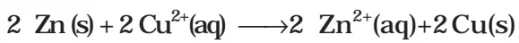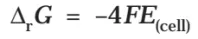If the concentration of all the reacting species is unity, then E(cell)
Thus, from the measurement of ECell we can obtain an important thermodynamic quantity, ΔrG, standard Gibbs energy of the reaction.From the latter we can calculate equilibrium constant by the equation: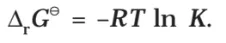Relationship between ΔG and electrode potential:

Let n, Faraday charge is taken out from a cell of e.m.f. (E) then electrical work done by the cell may be calculated as,

Work done = Charge × Potential = nFE

From thermodynamics we know that decrease in Gibbs free energy of a system is a measure of reversible or maximum obtainable work by the system if there is no work due to volume expansion

ΔG = - nFE

Under standard state ΔGº = - nFEº ............(1)

(i) From thermodynamics we know, DG = negative for spontaneous process. Thus from eq. (i) it is clear that the EMF should be ve for a cell process to be feasible or spontaneous.

(ii) When ΔG = positive, E = negative and the cell process will be non spontaneous.

 Reactions ΔG E Spontaneous (-) (+) Non-spontaneous (+) (-) Equilibrium 0 0

Standard free energy h, range of a cell may be calculated by electrode potential data.

Substituting the value of Eº (i.e., standard reduction potential of cathode-standard reduction potential of anode) in eq. (i) we may get ΔGº.

Measurement of Electrode Potential

Concept of electromotive force (EMF) of A Cell

Electron flows from anode to cathode in external circuit due to a pushing effect called or electromotive force (e.m.f.). EMF is called as cell potential. Unit of e.m.f. of cell is volt.

EMF of cell may be calculated as:

Ecell = reduction potential of cathode - Reduction potential of anode

Similarly, standard e.m.f. of the cell (Eº) may be calculated as

cell = Standard reduction potential of cathode - Standard reduction potential of anode.

Sign Convention of EMF

EMF of cell should be positive other wise it will not be feasible in the given direction.

Zn|ZnSO4||CuSO4|Cu   , E = 1.10 volt (Feasible)

Cu|CuSO4||ZnSO4|Zn,   E = - 1.10 volt (Not Feasible)

Salt Bridge

Two electrolyte solutions in galvanic cells are separated using salt bridge as represented in the Fig. Salt bridge is a device to minimize or eliminate the liquid junction potential. Saturated solution of salt like KCl, KNO3, NH4Cl and NH4NO3 etc. in agar-agar gel is used in salt bridge.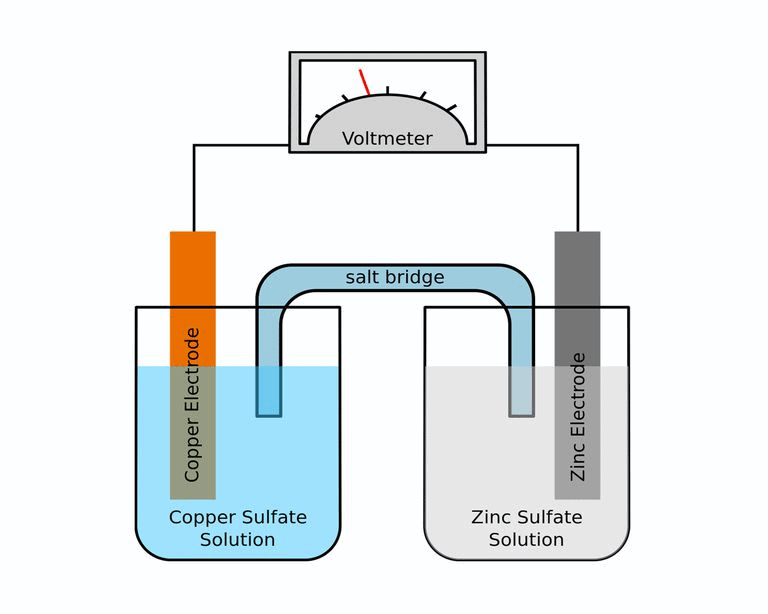Fig: Salt bridgeSalt bridge contains high concentration of ions viz. K+  and NO3at the junction with electrolyte solution. Thus, salt bridge carries whole of the current across the boundary; more over the K+  and NO3- ions have same speed. Hence, salt bridge with uniform and same mobility of cations and anions completes the electrical circuit & permits the ions to migrate.

Offer running on EduRev: Apply code STAYHOME200 to get INR 200 off on our premium plan EduRev Infinity!

## Chemistry for JEE

198 videos|450 docs|370 tests

,

,

,

,

,

,

,

,

,

,

,

,

,

,

,

,

,

,

,

,

,

;